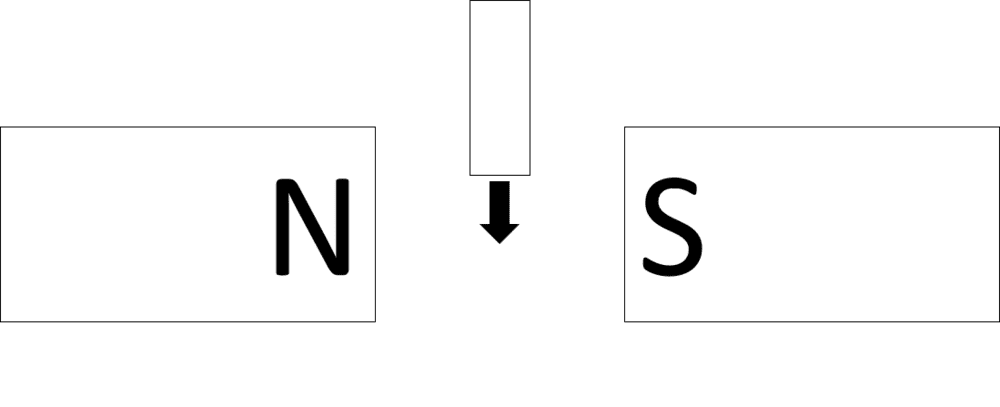# How to use Lenz' law

thebosonbreaker
I have been studying electromagnetic induction and have looked at the laws of Faraday and Lenz.

Faraday's law makes perfect sense to me, but I can't seem to grasp Lenz's law. I have read about it and watched many yt videos, and the idea seems simple. But actually using it to predict e.g. the direction of induced emf, I am having some trouble with.

Consider the following diagram. It shows a conductor being moved downwards through the poles of two magnets.The flux through the conductor is changing, so an emf is induced. Lenz's law tells us that: 'The direction of the induced emf is such as to oppose the change that caused it.'

As I understand it, this means that the current induced in the conductor will flow in a direction such that it creates a magnetic field which is opposing the CHANGE IN the external magnetic field (going L>R in the diagram between the magnets). This counteracts the downwards motion of the conductor. This makes sense.

But how do I actually work out the direction of this current? I don't understand how exactly the external field is "changing" as the conductor moves through it.

Could somebody please clarify this and talk through how they would work it out.

#### Attachments

Homework Helper
Gold Member
The induced EMF creates a current that has magnetic fields that are sort of given by the right hand rule=I like to think of it as a current into the paper creates a clockwise magnetic field. I don't use a right-hand rule, although many people do. These EMF's and currents must be such as to oppose the field of the permanent magnet. If you pick one direction as a guess, and check the magnetic field, and it points in the same direction as that of the permanent magnet, then you guessed wrong and it must be the other direction.
Edit: After reading @ZapperZ 's post, I realized that I forgot to mention one thing: For the case I did above, I'm referring to when you move the loop into the space between the two magnetic poles. If you then remove the loop from that space, the response is in the opposite direction.

Last edited:
•thebosonbreaker
Staff Emeritus
I have been studying electromagnetic induction and have looked at the laws of Faraday and Lenz.

Faraday's law makes perfect sense to me, but I can't seem to grasp Lenz's law.

That's a bit puzzling, because Lenz's Law is the qualitative description of Faraday's law.

Let's look at your example. The B-field is pointing to the right.

Case 1: Loop sliding towards the center in between the magnets.

Here, the flux is increasing, since the B-field is getting larger. Thus, an induced current will try to oppose this change in flux by generating an induced current such that the induced field will be in the opposite direction, i.e. to the left. So looking FROM the left side, you'll see a ccw current.

Case 2: Loop passed through the center and sliding away from in between the magnets.

Here, the flux is decreasing, since the B-field is getting smaller. The induced current now will try to set up an induced B field to oppose this decrease. And so, it will generating an induced B-field pointing to the right, in the same direction as the external B-field. Thus, the induced current in the loop will be cw when viewed from the left side.

The "minus" sign in Faraday's law is exactly what is going on here to match Lenz's law in terms of the direction of the induced emf and current.

Zz.

•Gold Member
The "minus" sign in Faraday's law is exactly what is going on here to match Lenz's law in terms of the direction of the induced emf and current.
+1
The logic of the situation is that it has to have the sign that it does. If the sign were different, there would be an infinite source of energy - just as with the Reactive Force in Newton's Third Law of Motion.

Homework Helper
Is that rectangle moving down a loop or just "a conductor", as described in the OP?

Staff Emeritus